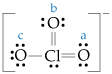# Problem: The Lewis structure for the chlorate ion is shown below. A. Calculate the formal charge on the chlorine (Cl) atom.B. Calculate the formal charge of each of the oxygen (O) atoms labeled a, b and c in the following Lewis structure.

87% (15 ratings)
###### Problem Details

The Lewis structure for the chlorate ion is shown below.

A. Calculate the formal charge on the chlorine (Cl) atom.

B. Calculate the formal charge of each of the oxygen (O) atoms labeled a, b and c in the following Lewis structure.What scientific concept do you need to know in order to solve this problem?

Our tutors have indicated that to solve this problem you will need to apply the Formal Charge concept. You can view video lessons to learn Formal Charge. Or if you need more Formal Charge practice, you can also practice Formal Charge practice problems.

What is the difficulty of this problem?

Our tutors rated the difficulty ofThe Lewis structure for the chlorate ion is shown below. A. ...as low difficulty.

How long does this problem take to solve?

Our expert Chemistry tutor, Dasha took 2 minutes and 42 seconds to solve this problem. You can follow their steps in the video explanation above.

What professor is this problem relevant for?

Based on our data, we think this problem is relevant for Professor Wolf's class at ASU.